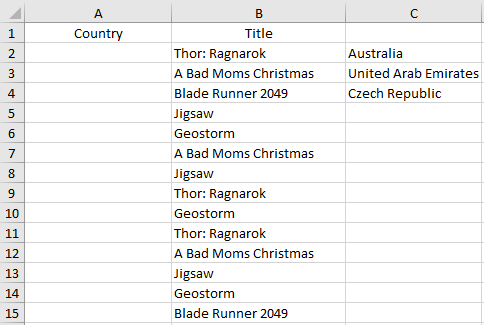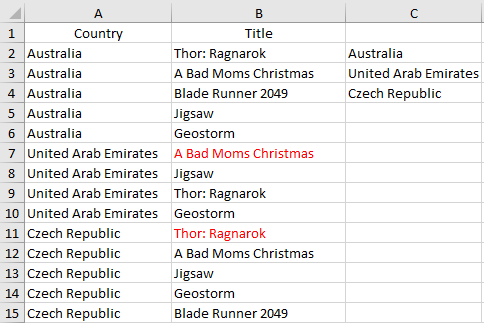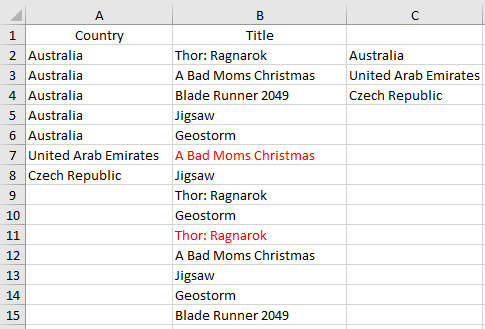Start the loop again when finding a duplicate in the same cell

I have a Excel file, its look like:After the macros, this will be the end results:I want macro that read column "B", and paste first country from column "C" in the same cells of column "A", after he find duplicate cell in "B", he copy next country from column "C", and does the same as I wrote with first country and etc.

I have a code:
``````Sub x()

Dim r1 As Long, r2 As Long

r1 = 2: r2 = 2

Do While Cells(r1, 2) <> vbNullString
If IsNumeric(Application.Match(Cells(r1, 2), Range(Cells(1, 2), Cells(r1 - 1, 2)), 0)) Then
r2 = r2 + 1
End If
Cells(r1, 1).Value = Cells(r2, 3).Value
r1 = r1 + 1
Loop

End Sub
``````

But it does not work as it should:I want when macro find first duplicate in column "B" (in my case B7 "A Bad Moms Christmas") he starting find duplicate from this cell and not paying attention to past cells, and paste same country from "C" (United Arab Emirates), before he find another duplicate and etc.
Can someone help? Thanks.
Who is Participating?
I wear a lot of hats...

"The solutions and answers provided on Experts Exchange have been extremely helpful to me over the last few years. I wear a lot of hats - Developer, Database Administrator, Help Desk, etc., so I know a lot of things but not a lot about one thing. Experts Exchange gives me answers from people who do know a lot about one thing, in a easy to use platform." -Todd S.

ConsultantCommented:
Hi Alex,

Can you post a sample file rather than us having to re-create it from scratch.

Also, does it have to be VBA?  A formula might be easier, and has the advantage of being self-documenting, and not requiring an XLSM file.

Alan.
Author Commented:
Hi Alan.
I attach file. Yes it must be VBA.
data.xlsm
ConsultantCommented:
Hi Alex,

Try this:

``````Sub x()

Dim r1 As Long, r2 As Long, r3 As Long

r1 = 2: r2 = 2: r3 = 1

Do While Cells(r1, 2) <> vbNullString
If IsNumeric(Application.Match(Cells(r1, 2), Range(Cells(r3, 2), Cells(r1 - 1, 2)), 0)) Then
r2 = r2 + 1
r3 = r1
End If
Cells(r1, 1).Value = Cells(r2, 3).Value
r1 = r1 + 1

Loop

End Sub
``````

Alan.

Experts Exchange Solution brought to you by